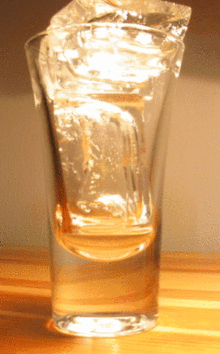# 12.1: Interactions between Molecules

$$\newcommand{\vecs}{\overset { \rightharpoonup} {\mathbf{#1}} }$$ $$\newcommand{\vecd}{\overset{-\!-\!\rightharpoonup}{\vphantom{a}\smash {#1}}}$$$$\newcommand{\id}{\mathrm{id}}$$ $$\newcommand{\Span}{\mathrm{span}}$$ $$\newcommand{\kernel}{\mathrm{null}\,}$$ $$\newcommand{\range}{\mathrm{range}\,}$$ $$\newcommand{\RealPart}{\mathrm{Re}}$$ $$\newcommand{\ImaginaryPart}{\mathrm{Im}}$$ $$\newcommand{\Argument}{\mathrm{Arg}}$$ $$\newcommand{\norm}{\| #1 \|}$$ $$\newcommand{\inner}{\langle #1, #2 \rangle}$$ $$\newcommand{\Span}{\mathrm{span}}$$ $$\newcommand{\id}{\mathrm{id}}$$ $$\newcommand{\Span}{\mathrm{span}}$$ $$\newcommand{\kernel}{\mathrm{null}\,}$$ $$\newcommand{\range}{\mathrm{range}\,}$$ $$\newcommand{\RealPart}{\mathrm{Re}}$$ $$\newcommand{\ImaginaryPart}{\mathrm{Im}}$$ $$\newcommand{\Argument}{\mathrm{Arg}}$$ $$\newcommand{\norm}{\| #1 \|}$$ $$\newcommand{\inner}{\langle #1, #2 \rangle}$$ $$\newcommand{\Span}{\mathrm{span}}$$$$\newcommand{\AA}{\unicode[.8,0]{x212B}}$$

In the winter, many people find the snow and ice beautiful; they enjoy getting out to ski or ice-skate. When the snow melts, however, the roads get very sloppy and messy. Some people look forward to spring, when the ice and snow are gone and the weather is warmer. All of these events and factors are dependent on the melting point of a solid and the freezing point of a liquid.

## Melting Point

Solids are similar to liquids in that both are condensed states, with particles that are far closer together than those of a gas. However, while liquids are fluid, solids are not. The particles of most solids are packed tightly together in an orderly arrangement. The motion of individual atoms, ions, or molecules in a solid is restricted to vibrational motion about a fixed point. Solids are almost completely incompressible and are the densest of the three states of matter.

As a solid is heated, its particles vibrate more rapidly as it absorbs kinetic energy. Eventually, the organization of the particles within the solid structure begins to break down and the solid starts to melt. The melting point is the temperature at which a solid changes into a liquid. At its melting point, the disruptive vibrations of the particles of the solid overcome the attractive forces operating within the solid. As with boiling points, the melting point of a solid is dependent on the strength of those attractive forces. Sodium chloride $$\left( \ce{NaCl} \right)$$ is an ionic compound that consists of a multitude of strong ionic bonds. Sodium chloride melts at $$801^\text{o} \text{C}$$. Ice (solid $$\ce{H_2O}$$) is a molecular compound of molecules that are held together by hydrogen bonds. Though hydrogen bonds are the strongest of the intermolecular forces, the strength of hydrogen bonds is much less than that of ionic bonds. The melting point of ice is 0 °C.Figure $$\PageIndex{1}$$: Melting ice cubes illustrate the process of this phase transition. (Public Domain; Moussa).

The melting point of a solid is the same as the freezing point of the liquid. At that temperature, the solid and liquid states of the substance are in equilibrium. For water, this equilibrium occurs at $$0^\text{o} \text{C}$$.

$\ce{H_2O} \left( s \right) \rightleftharpoons \ce{H_2O} \left( l \right) \nonumber$

We tend to think of solids as those materials that are solid at room temperature. However, all materials have melting points of some sort. Gases become solids at extremely low temperatures, and liquids will also become solid if the temperature is low enough. The table below gives the melting points of some common materials.

Materials Melting Point (°C)
Table $$\PageIndex{1}$$:Melting Points of Common Materials
Hydrogen -259
Oxygen -219
Diethyl ether -116
Ethanol -114
Water 0
Pure silver 961
Pure gold 1063
Iron 1538

## Summary

The melting point is the temperature at which a solid changes into a liquid. Intermolecular forces have a strong influence on melting point.

12.1: Interactions between Molecules is shared under a not declared license and was authored, remixed, and/or curated by LibreTexts.